### Still have math questions?

Scientific Notation Length Conversion Area Conversion

### Calculator

Area
Perimeter
Square
Rectangle
Triangle
Circle
Parallelogram
Trapezoid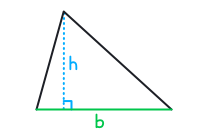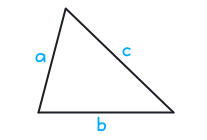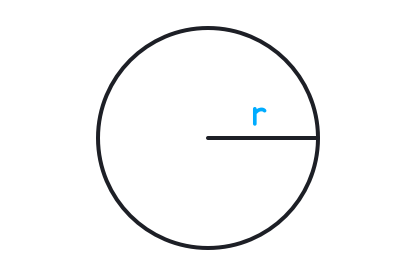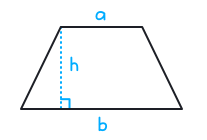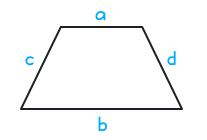Area = a2
a
cm
Area = cm2

### Knowledge

Area (A) is measured in square units, perimeter (P) is measured in units, and circumference (C) is measured in units.

#### SquareP = 4a

A = a2

#### RectangleP = 2a+2b

A = ab

#### ParallelogramP = 2a+2b

A = bh

#### TrapezoidP = a+b+c+d

A = 12h(a+b)

#### Triangle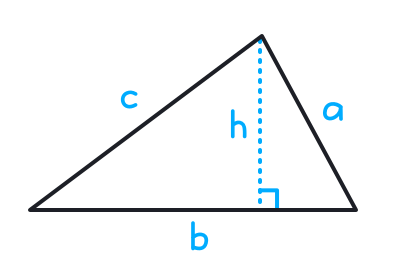P = a+b+c

A = 12bh

#### CircleC = 2πr

A = πr2

Please provide numbers only. Please provide at least two numbers. Please provide 2 to 10 non-zero integer numbers. None